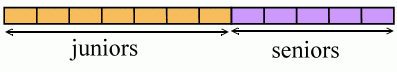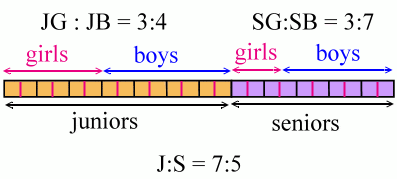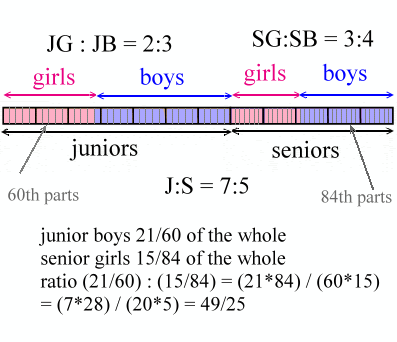### A bar diagram to solve a ratio problem

Dave at MathNotations had an interesting ratio problem:

In Virtual HS, the ratio of the number of juniors to seniors is 7:5.
The ratio of (the number of) junior males to junior females is 3:2.
The ratio of senior males to senior females is 4:3.
What is the ratio of junior males to senior females?

He asked if it can be solved using "Singapore" style bar model.

I'm not sure if this is exactly how they'd do it, but this is how I'd do it... so here goes.

After I made the diagrams, I soon saw that Dave's numbers are two awkward; the bar diagram drawing would get too messy because we'd need to divide it into too tiny parts to see anything.

BUT... you probably know about the PROBLEM SOLVING STRATEGY called "solve an easier problem". My agenda is therefore:

• show how to solve a few related simple ratio problems using the bar diagram

• solve a variant of the original problem (with friendly numbers)

• solve the original problem.

1. Here's a bar diagram representing the fact that the ratio of the number of juniors to seniors is 7:5.As you can see, the "whole" ends up divided into 12 parts (7 + 5).

We can use this diagram to solve problems such as:

If there are 768 juniors and seniors in total, how many juniors are in the school?
(Divide that into 12 parts, and then take 7 of those parts.)

Or...

If there are 95 seniors, how many juniors are there?
(Divide the number of seniors by 5, then multiply by 7.)

Or..
If there are 49 Juniors, how many students are there in all?
(Divide the number of juniors by 7, then multiply by 12.)

The diagram makes all this dividing/multiplying by 5/7/12 all crystal clear.

2. Let's change the numbers from the original problem to make them "friendly" for this approach. Let's solve this instead:

In Virtual HS, the ratio of the number of juniors to seniors is 7:5.
The ratio of (the number of) junior males to junior females is 4:3.
The ratio of senior males to senior females is 7:3.
What is the ratio of junior males to senior females?

Our diagram becomes, first of all, like before:But then we add the additional information into it. The ratio of junior males to females is 4:3, which means juniors as a whole are divided into 7 parts (4 + 3). Similarly, seniors need divided into 10 parts.

Voila! It just so happens (wonder why?) that our original division into 7 and 5 parts works beautifully to give us juniors divided into 7 parts (they already are!) and seniors into 10 parts if we only split each part into 2.So, what is the ratio of junior males to senior females?

In the diagram, the WHOLE is now divided into 24 parts. Junior males are 8 of those parts, and senior females are 3 of those parts. Their ratio is therefore 8:3.

3. Solving the original problem, with those unfriendly numbers.... the diagram looks sort of like this:It gets messy... I'd prefer using the bar model as a stepping stone and to illustrate the basic situation, but eventually using the "least common denominator" or algebraic methods.Dave Marain said…
Teach me those graphics, Maria! Luv it! And thanks for the kind reference to my post...
Very clear explanation. Ironically, I outlined a 'make it simpler' approach in one of the comments to my post. I'd be interested in your take on the multiplication of ratios idea, both numerically and symbolically. appropriate for middle schoolers? I think so but I value your opinion.Maria Miller said…
The multiplication of ratios idea is just really efficient! I figure you could teach it to middle schoolers; however I suppose they don't usually do many such problems that would require it, right?karrelbarrel said…
I was wondering if you could do it this way....

If you use a model similar to the "Singapore" model, called "thinking blocks",

Let me see if I can set it up here:

I'm going to use some user friendly numbers,

Let's say there are 490 Juniors

and 350 Seniors ( a ratio of 7:5)

Juniors: 70 70 70 70 70 70 70 = 490

Seniors: 70 70 70 70 70 = 350

Also, the Junior group can be divided into 5 groups showing your male to female ratio of 3:2, and the Seniors can be divided into 7 groups showing the 4:3 ratio:

Juniors: 70 70 70 70 70 70 70
98 98 98 98 98

Seniors: 70 70 70 70 70
50 50 50 50 50 50 50

So the ratio of Junior males (98x3)
to Senior females (50x3) is

294:150 or 49:25

I think this graphic solution works with very little calculation

I've been trying to get my students to look at things more visually.